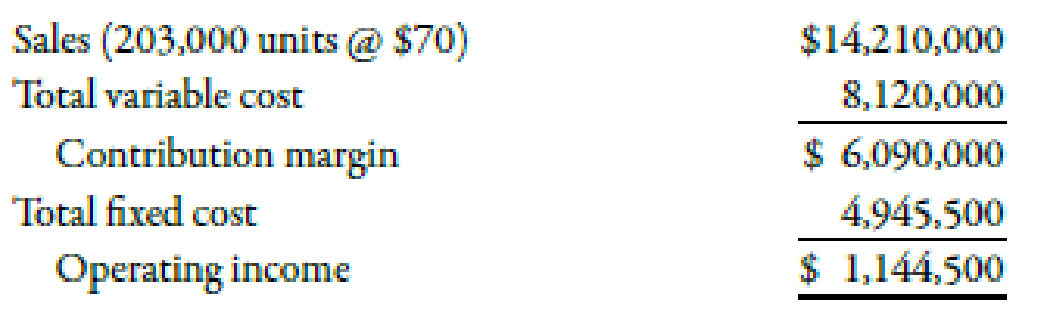Chapter 7, Problem 53P### Managerial Accounting: The Corners...

7th Edition
Maryanne M. Mowen + 2 others
ISBN: 9781337115773

#### Solutions

Chapter
Section### Managerial Accounting: The Corners...

7th Edition
Maryanne M. Mowen + 2 others
ISBN: 9781337115773
Textbook Problem
12 views

# Aldovar Company produces a variety of chemicals. One division makes reagents for laboratories. The division’s projected income statement for the coming year is:Required: 1. Compute the contribution margin per unit, and calculate the break-even point in units. (Note: Round answer to the nearest unit.) Calculate the contribution margin ratio and use it to calculate the break-even sales revenue. (Note: Round contribution margin ratio to four decimal places, and round the break-even sales revenue to the nearest dollar.) 2. The divisional manager has decided to increase the advertising budget by $250,000. This will increase sales revenues by$1 million. By how much will operating income increase or decrease as a result of this action? 3. Suppose sales revenues exceed the estimated amount on the income statement by $1,500,000. Without preparing a new income statement, by how much are profits underestimated? 4. Compute the margin of safety based on the original income statement. 5. Compute the degree of operating leverage based on the original income statement. If sales revenues are 8% greater than expected, what is the percentage increase in operating income? (Note: Round operating leverage to two decimal places.) 1. To determine Calculate contribution margin per unit, break-even point in units, contribution margin ratio and break-even sales revenue. Explanation Contribution margin: Contribution margin can be defined as the amount obtained after deducting the variable expense from sales revenue. It means the amount of sales left after covering the variable expenses. Break-Even Point: The point or situation where the amount of total cost is equivalent to total revenue is known as the break-even point. It is the point where there is no loss or no profit. Contribution Margin Ratio: The sales percentage remaining after covering the amount of total variable cost is known as the contribution margin ratio. It is the available sales dollar percentage which will be used to cover the total fixed cost. Break-Even Sales Revenue: Break-even sales revenue can be evaluated by dividing the total amount of fixed cost by the contribution margin ratio. Use the following formula to calculate contribution margin: Contributionmarginperunit=ContributionmarginUnitssold Substitute$6,090,000 for contribution margin and 203,000 for units sold in the above formula.

Contributionmarginperunit=$6,090,000203,000=$30

Therefore, the contribution margin per unit is $30. Use the following formula to calculate break-even units: Break-even units=Total fixed costContribution marginperunit Substitute$4,945,500 for total fixed cost and \$30 for contribution margin per unit in the above formula

2.

To determine

Calculate the effect of increase of in advertising budget and sales revenues on the operating income.

3.

To determine

Find out the amount of underestimated profit due to increase in estimated amount of sales.

4.

To determine

Calculate margin of safety on the basis of original information.

5.

To determine

Calculate the degree of operating leverage on the basis of original information. Also calculate the percentage increase in operating income if there is increase of 8% in the expected sales revenues.

### Still sussing out bartleby?

Check out a sample textbook solution.

See a sample solution

#### The Solution to Your Study Problems

Bartleby provides explanations to thousands of textbook problems written by our experts, many with advanced degrees!

Get Started

#### Is a physical inventory necessary under the perpetual system? Why or why not?

College Accounting, Chapters 1-27 (New in Accounting from Heintz and Parry)

#### Next, we need to calculate MMMs cost of debt. We can use different approaches to estimate it One approach is to...

Fundamentals of Financial Management, Concise Edition (with Thomson ONE - Business School Edition, 1 term (6 months) Printed Access Card) (MindTap Course List)

#### What is the purpose of a base period?

Cornerstones of Cost Management (Cornerstones Series)